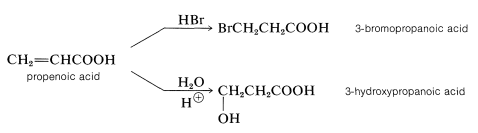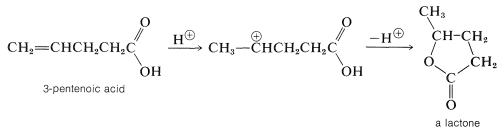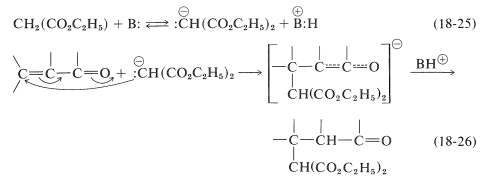# 18.10: Reactions of Unsaturated Carboxylic Acids and Their Derivatives

$$\newcommand{\vecs}{\overset { \rightharpoonup} {\mathbf{#1}} }$$ $$\newcommand{\vecd}{\overset{-\!-\!\rightharpoonup}{\vphantom{a}\smash {#1}}}$$$$\newcommand{\id}{\mathrm{id}}$$ $$\newcommand{\Span}{\mathrm{span}}$$ $$\newcommand{\kernel}{\mathrm{null}\,}$$ $$\newcommand{\range}{\mathrm{range}\,}$$ $$\newcommand{\RealPart}{\mathrm{Re}}$$ $$\newcommand{\ImaginaryPart}{\mathrm{Im}}$$ $$\newcommand{\Argument}{\mathrm{Arg}}$$ $$\newcommand{\norm}{\| #1 \|}$$ $$\newcommand{\inner}{\langle #1, #2 \rangle}$$ $$\newcommand{\Span}{\mathrm{span}}$$ $$\newcommand{\id}{\mathrm{id}}$$ $$\newcommand{\Span}{\mathrm{span}}$$ $$\newcommand{\kernel}{\mathrm{null}\,}$$ $$\newcommand{\range}{\mathrm{range}\,}$$ $$\newcommand{\RealPart}{\mathrm{Re}}$$ $$\newcommand{\ImaginaryPart}{\mathrm{Im}}$$ $$\newcommand{\Argument}{\mathrm{Arg}}$$ $$\newcommand{\norm}{\| #1 \|}$$ $$\newcommand{\inner}{\langle #1, #2 \rangle}$$ $$\newcommand{\Span}{\mathrm{span}}$$$$\newcommand{\AA}{\unicode[.8,0]{x212B}}$$

Unsaturated carboxylic acids of the type $$\ce{RCH=CH(CH_2)}_n \ce{COOH}$$ usually exhibit the properties characteristic of isolated double bonds and isolated carboxyl groups when $$n$$ is large and the functional groups are far apart. As expected, exceptional behavior is found most commonly when the groups are sufficiently close together to interact strongly, as in $$\alpha$$,$$\beta$$-unsaturated acids, $$\ce{R} \overset{\beta}{\ce{C}} \ce{H=} \overset{\alpha}{\ce{C}} \ce{CO_2H}$$. We shall emphasize those properties that are exceptional in the following discussion.

## Migration of the Double Bond

In the presence of strong base, $$\alpha$$,$$\beta$$- and $$\beta$$,$$\gamma$$-unsaturated carboxylic acids tend to interconvert by migration of the double bond:Ester derivatives, $$\ce{RCH=CH-CH_2COOR'}$$, and the corresponding unsaturated aldehydes and ketones, $$\ce{RCH=CH-CH_2COR'}$$, are much more prone to this type of rearrangement than are the acids.

## Hydration and Hydrogen Bromide Addition

Like alkenes, the double bonds of $$\alpha$$,$$\beta$$-unsaturated acids can be brominated, hydroxylated, hydrated, and hydrobrominated, although the reactions often are relatively slow. In the addition of unsymmetrical reagents the direction of addition is opposite to that observed for alkenes (anti-Markovnikov). Thus propenoic (acrylic) acid adds hydrogen bromide and water to form 3-bromo- and 3-hydroxypropanoic acids:These additions are analogous to the addition of halogens and halogen acids to 1,3-butadiene (Section 13-2). In the first step, a proton is transferred to the carbonyl oxygen. The resulting conjugate acid can be regarded as a resonance hybrid of structures $$16a$$-$$16d$$:In the second step, a nucleophile (such as $$\ce{Br}^\ominus$$ or a water molecule) attacks an electron-deficient carbon of the hybrid $$16$$. Attack at the carboxyl carbon may occur but does not lead to a stable product. Attack of the nucleophile at the $$\beta$$ carbon, however, produces the enol form of the $$\beta$$-substituted acid, which then is converted rapidly to the normal carboxylic acid:## Lactone Formation

When the double bond of an unsaturated acid is farther down the carbon chain than between the alpha and beta positions, the so-called "conjugate addition" is not possible. Nonetheless, the double bond and carboxyl group frequently interact in the presence of acidic catalysts because the carbocation that results from addition of a proton to the double bond has a built-in nucleophile (the carboxyl group), which may attack the cationic center to form a cyclic ester called a lactone.

Lactone formation only occurs readily by this mechanism when a five- or six-membered ring can be formed:Five- or six-membered lactones also are formed by internal esterification when either $$\gamma$$- or $$\delta$$-hydroxy acids are heated. Under similar conditions, $$\beta$$-hydroxy acids are dehydrated to $$\alpha$$,$$\beta$$-unsaturated acids, whereas $$\alpha$$-hydroxy acids undergo bimolecular esterification to substances with six-membered dilactone rings called lactides:## More on the Michael Addition

The foregoing examples of addition to the double bonds of unsaturated carboxylic acids all involve activation by an electrophilic species such as $$\ce{H}^\oplus$$. Conjugate addition also may occur by nucleophilic attack on acid derivatives, the most important being the base-catalyzed Michael addition (Section 17-5B) and 1,4-addition of organometallic compounds (Section 14-12D). In all of these reactions a nucleophilic agent, usually a carbanion, attacks the double bond of an $$\alpha$$,$$\beta$$-unsaturated compound in which the double bond is conjugated with, and activated by, a strongly electronegative unsaturated group (such as $$\ce{-CN}$$, $$\ce{-NO_2}$$, etc.). In the Michael addition, the carbanion usually is an enolate salt.

The overall reaction is illustrated here by the specific example of the addition of diethyl propanedioate (diethyl malonate) to ethyl 3-phenylpropenoate (ethyl cinnamate):The mechanism of this kind of transformation, with diethyl propanedioate as the addend, is outlined in Equations 18-25 and 18-26. The basic catalyst required for the Michael addition (here symbolized as $$\ce{B:}$$) serves by forming the corresponding anion:A variety of nucleophilic agents can be used; propanedinitrile, 3-oxobutanoate esters, and cyanoethanoate esters all form relatively stable carbanions and function well in Michael addition reactions. Obviously, if the carbanion is too stable, it will have little or no tendency to attack the double bond of the $$\alpha$$,$$\beta$$-unsaturated acid derivative.

Enamines (Sections 16-4C and 17-4B) are excellent addends in many Michael-type reactions. An example is provided by the addition of $$\ce{N}$$-(1-cyclohexenyl)-azacyclopentane to methyl 2-methylpropanoate:## Contributors and Attributions

John D. Robert and Marjorie C. Caserio (1977) Basic Principles of Organic Chemistry, second edition. W. A. Benjamin, Inc. , Menlo Park, CA. ISBN 0-8053-8329-8. This content is copyrighted under the following conditions, "You are granted permission for individual, educational, research and non-commercial reproduction, distribution, display and performance of this work in any format."

This page titled 18.10: Reactions of Unsaturated Carboxylic Acids and Their Derivatives is shared under a not declared license and was authored, remixed, and/or curated by John D. Roberts and Marjorie C. Caserio.